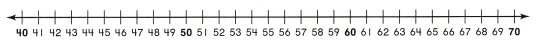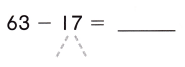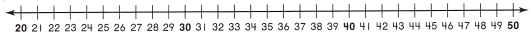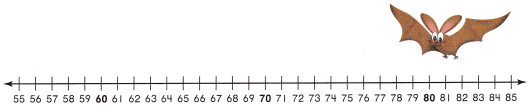# Texas Go Math Grade 2 Lesson 8.2 Answer Key Break Apart Numbers to Subtract

Refer to our Texas Go Math Grade 2 Answer Key Pdf to score good marks in the exams. Test yourself by practicing the problems from Texas Go Math Grade 2 Lesson 8.2 Answer Key Break Apart Numbers to Subtract.

## Texas Go Math Grade 2 Lesson 8.2 Answer Key Break Apart Numbers to Subtract

Problem Solving

Break apart the number you are subtracting. Write the difference.Question 5.57 – 15 = 42
Explanation:
15 is written as 7 and 8
57 – 7 = 50
50 – 8 = 42

Question 6.63 – 17 = 46
Explanation:
17 is written as 3 and 14
63 – 3 = 60
60 – 14 = 46

Question 7.
68 – 19 = __________
68 – 19 = 49
Explanation:
19 can be written as 8 and 11
68 – 8 = 60
60 – 11 = 49

Question 8.
61 – 18 = ___________
61 – 18 = 43
Explanation:
18 is written as 1 and 17
61 – 1 = 60
60 – 17 = 43

Solve. Write or draw to explain.

Question 9.
H.O.T. Jane has 53 toys in a box. She takes some toys out. Now there are 36 toys in the box. How many toys did Jane take out of the box?__________ toys
17 toys
Explanation:
Jane has 53 toys in a box.
She takes some toys out.
Now there are 36 toys in the box.
53 – 36 = 17
17 toys that Jane take out of the box

Question 10.
H.O.T. Multi-Step Look at Tomes steps to solve a problem. Solve this problem in the same way.
42 – 15 = ?42 – 15 = 27
42 – 10 = 32
32 – 2 = 30
30 – 3 = 27
Explanation:
15 is written as 10 + 2 + 3

Question 11.
Use Tools 42 people can visit a cave at one time. 16 people are already in the cave. How many more people con enter?(A) 32
(B) 24
(C) 26
Explanation:
2 people can visit a cave at one time. 16 people are already in the cave.
42 – 16 = 26
26 more people con enter

Question 12.
Multi-Step There are 76 bats in a cave. 23 of the bats flew out. Then 4 bats flew into the cave. How many bats are in the cave?(A) 59
(B) 60
(C) 57
Explanation:
There are 76 bats in a cave.
23 of the bats flew out.
Then 4 bats flew into the cave.
76 – 23 = 53
53 + 4 = 57
57 bats are in the cave

Question 13.
TEXAS Test Prep There are 32 papers in the first stack. There are 19 papers in the second stack. How many more papers are in the first stock than in the second stack?(A) 23 more
(B) 51 more
(C) 13 more
Explanation:
There are 32 papers in the first stack.
There are 19 papers in the second stack.
32 – 19 = 13
13 more papers are in the first stock than in the second stack

TAKE HOME ACTIVITY • Ask your child to write o subtraction story that uses 2-digit numbers.